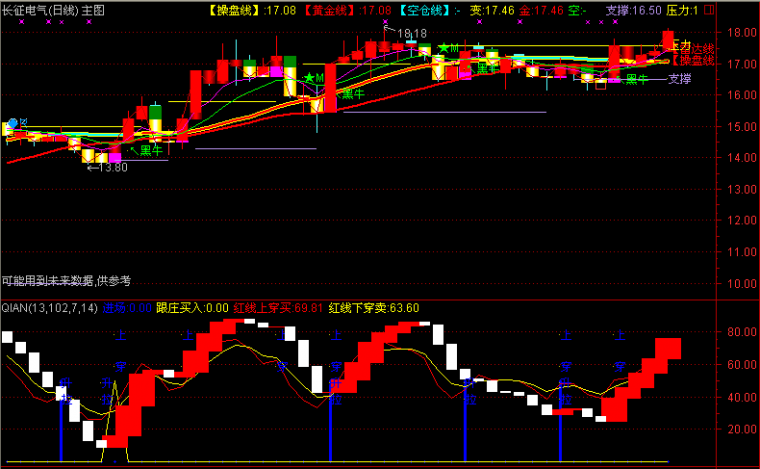﻿ 史上最简单的指标公式“一条线”-大智慧公式 －程序化交易（CXH99.COM）

# 史上最简单的指标公式“一条线”[大智慧公式]

来源:程序化99（ WWW.CXH99.COM ）

V1:=(C*2+H+L)/4*10;
V2:=EMA(V1,13)-EMA(V1,34);
V3:=EMA(V2,5);
V4:=2*(V2-V3)*5.5;

V5:=(HHV(INDEXH,8)-INDEXC)/(HHV(INDEXH,8)-LLV(INDEXL,8))*8;
V6:=EMA(3*V5-2*SMA(V5,18,1),5);
V7:=(INDEXC-LLV(INDEXL,8))/(HHV(INDEXH,8)-LLV(INDEXL,8))*10;
V8:=(INDEXC*2+INDEXH+INDEXL)/4;
V9:=EMA(V8,13)-EMA(V8,34);
VA:=EMA(V9,3);
VB:=(V9-VA)/2;
0,COLORblue,LINETHICK2;【字体： 】【打印文章】【查看评论

没有相关内容